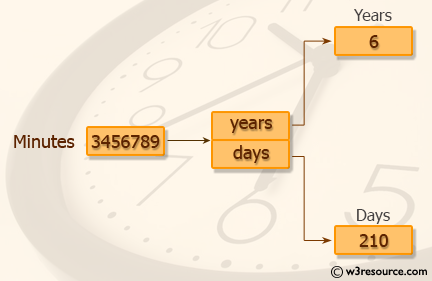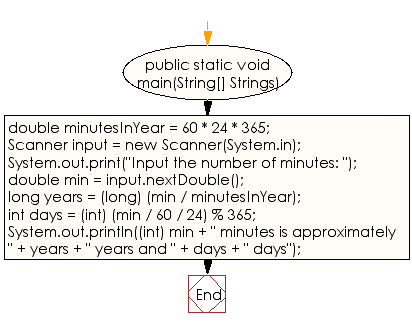﻿ Java exercises: Takes the user to input the minutes, and print the number of years and days for the minutes - w3resource# Java Data Type Exercises: Takes the user to input the minutes, and print the number of years and days for the minutes

## Java Data Type: Exercise-4 with Solution

Write a Java program to convert minutes into a number of years and days.

Test Data
Input the number of minutes: 3456789Sample Solution:

Java Code:

``````import java.util.Scanner;
public class Exercise4 {

public static void main(String[] Strings) {

double minutesInYear = 60 * 24 * 365;

Scanner input = new Scanner(System.in);

System.out.print("Input the number of minutes: ");

double min = input.nextDouble();

long years = (long) (min / minutesInYear);
int days = (int) (min / 60 / 24) % 365;

System.out.println((int) min + " minutes is approximately " + years + " years and " + days + " days");
}
}
```
```

Sample Output:

```Input the number of minutes: 3456789
3456789 minutes is approximately 6 years and 210 days
```

Flowchart:Java Code Editor :

Improve this sample solution and post your code through Disqus

What is the difficulty level of this exercise?

﻿

## Java: Tips of the Day

Checks if a string is upper case:

```public static boolean isUpperCase(String input) {
return Objects.equals(input, input.toUpperCase());
}
```

Ref: https://bit.ly/39Hpo84